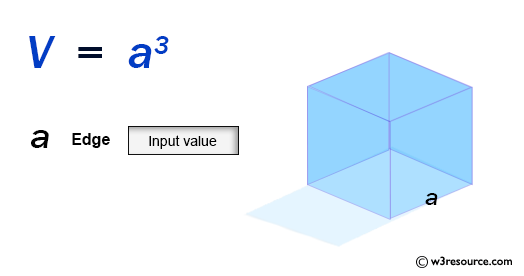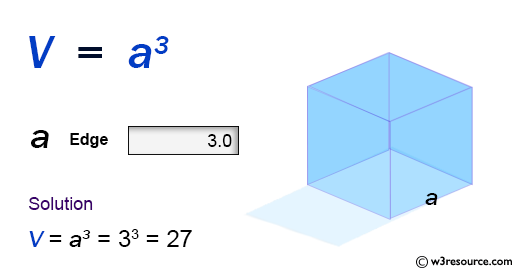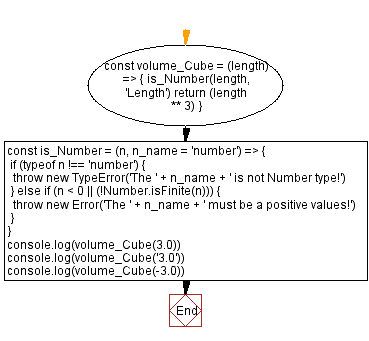# JavaScript: Volume of a Cube

## JavaScript Math: Exercise-57 with Solution

Write a JavaScript program to calculate the volume of a Cube.

From Wikipedia –
In geometry, a cube is a three-dimensional solid object bounded by six square faces, facets or sides, with three meeting at each vertex. The cube is the only regular hexahedron and is one of the five Platonic solids. It has 6 faces, 12 edges, and 8 vertices.

Sample Data:Sample Solution:

HTML Code:

``````<!DOCTYPE html>
<html>
<meta charset="utf-8">
<title>JavaScript function to Volume of a Cube</title>
<body>

</body>
</html>
```
```

JavaScript Code:

``````const volume_Cube = (length) => {
is_Number(length, 'Length')
return (length ** 3)
}
const is_Number = (n, n_name = 'number') => {
if (typeof n !== 'number') {
throw new TypeError('The ' + n_name + ' is not Number type!')
} else if (n < 0 || (!Number.isFinite(n))) {
throw new Error('The ' + n_name + ' must be a positive values!')
}
}
console.log(volume_Cube(3.0))
console.log(volume_Cube('3.0'))
console.log(volume_Cube(-3.0))
```
```

Sample Output:

```27
-------------------------------------------------
Uncaught TypeError: The Length is not Number type!
at https://cdpn.io/cpe/boomboom/pen.js?key=pen.js-02d7f226-5e95-ffb9-1102-3d13c98d5a6e:7
----------------------------------------------------------------------
Uncaught Error: The Length must be a positive values!
at https://cdpn.io/cpe/boomboom/pen.js?key=pen.js-e084be27-1a02-808e-2e24-5725028ec78d:9
```

Flowchart:Live Demo:

See the Pen javascript-math-exercise-57 by w3resource (@w3resource) on CodePen.

Improve this sample solution and post your code through Disqus

Previous: Volume of a Cuboid.
Next: Volume of a Cone.

What is the difficulty level of this exercise?

Test your Programming skills with w3resource's quiz.

﻿